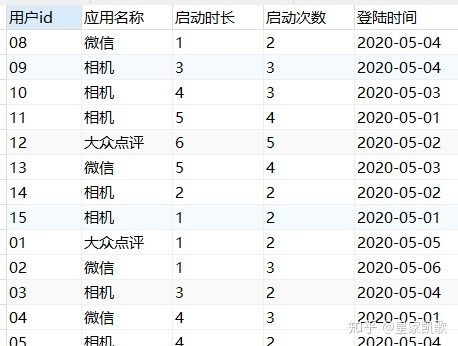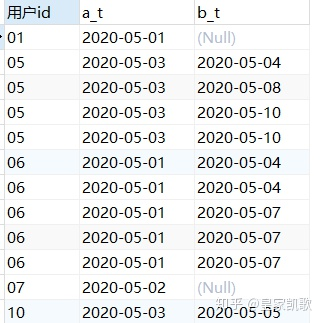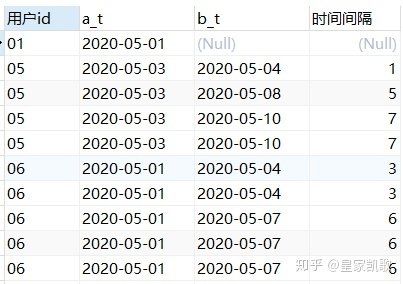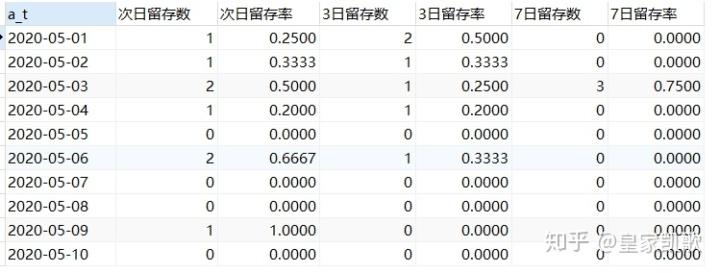# 活跃用户数怎么计算_留存率计算【面试题】N日活跃用户数，某日活跃的用户数在之后的第N日活跃用户数。

N日活跃留存率，N日留存用户数/某日活跃用户数

select a.用户id, a_t, b_t from
(select 用户id, 登陆时间 a_t from 留存率题目
where 应用名称 = '相机') a left join
(select 用户id, 登陆时间 b_t from 留存率题目
where 应用名称 = '相机') b
on a.用户id = b.用户id and a_t < b_tselect *, datediff(b_t, a_t) as 时间间隔 from
(select a.用户id, a_t, b_t from
(select 用户id, 登陆时间 a_t from 留存率题目
where 应用名称 = '相机') a left join
(select 用户id, 登陆时间 b_t from 留存率题目
where 应用名称 = '相机') b
on a.用户id = b.用户id and a_t < b_t) cselect a_t, count(distinct case when 时间间隔=1 then 用户id else null end) 次日留存数,
count(distinct case when 时间间隔=1 then 用户id else null end)/count(distinct 用户id) 次日留存率,
count(distinct case when 时间间隔=3 then 用户id else null end) 3日留存数,
count(distinct case when 时间间隔=3 then 用户id else null end)/count(distinct 用户id) 3日留存率,
count(distinct case when 时间间隔=7 then 用户id else null end) 7日留存数,
count(distinct case when 时间间隔=7 then 用户id else null end)/count(distinct 用户id) 7日留存率
from
(select *, datediff(b_t, a_t) as 时间间隔 from
(select a.用户id, a_t, b_t from
(select 用户id, 登陆时间 a_t  from 留存率题目
where 应用名称 = '相机') a left join
(select 用户id, 登陆时间 b_t from 留存率题目
where 应用名称 = '相机') b
on a.用户id = b.用户id and a_t < b_t) c) d
group by a_t2、时间间隔的计算，datediff和timestampdiff的用法区别；

3、分组计算case when的用法。

## 参考

1. ^注意datediff的格式为datediff（a,b），输出的是a-b的结果；此处也可以使用timestampdiff(day,a,b),输出结果为b-a。此外，datediff只用来计算以天为单位的时间间隔，timestampdiff则可以计算month/day/hour/second等时间间隔
11-30136812-15570
12-07433
12-15613
12-10254
07-212001
07-271288
12-151036
12-22708
11-105667
12-15142
01-101691
07-27456
03-011万+
10-165352
10-151万+
10-16324
©️2020 CSDN 皮肤主题: 1024 设计师:白松林